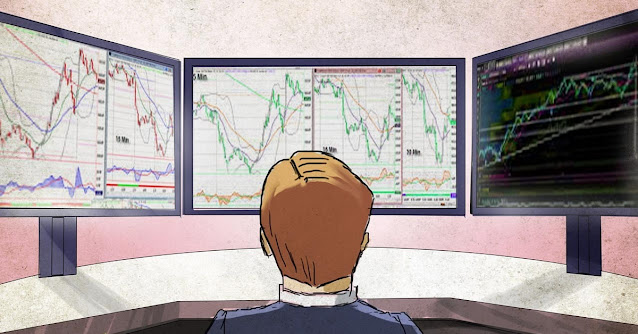Secret 1: The goal of the reversal/retracement and divergence

Few traders know that moving averages are the target of corrections or reversals when divergences occur.

That is when a divergence shows that the market can reverse. There will be traders thinking about where that reversal or pullback is going. Then the main moving average is targets good for this case.

For reversal traders, they can find a reasonable take profit point. For trend traders, they can know when to exit.

Of course, there will be cases where the price crosses the moving average, but we can judge that based on price action.

Secret 2: The speed and gravity of the average line

When humans reach a certain speed, we can temporarily jump off the ground despite the effects of gravity. When we lose that speed, gravity will pull us back to Earth. The higher we have the speed, the farther and higher we can jump.

We can also visualize the similarly correlated price and moving averages. As shown below:

As the price gains additional momentum (or speed), the price can move away from the moving averages before being pulled back by gravity from the moving average. When the price has a little momentum, it cannot go as far, as it will be pulled back by the gravity of the moving averages.

The angle of the 2 moving averages, the distance between them, will tell us whether the price has strong momentum to break out of the moving average. To determine the momentum from the moving average, we can use the moving average from period 5 to a maximum of 40.

For example EMA 5 and 10, or EMA 10 and 20 or EMA 20 and 40. The distance between two EMA lines will tell us the price momentum.

Secret # 3: EMA helps traders to quickly identify support resistance

To see if the moving average's gravity is working well, we can check the angle of the moving averages.

Moving averages between 30 and 80 are considered to be good EMAs. As shown below:

If the moving averages are flat, the gravity is very low. The price will be difficult to find back the average line.

So, when a moving average is doing well will have a slope above 30 degrees, we can use the moving average as a dynamic resistance or support, where when the price finds it, we will find opportunities to trade in trends.

▶️Telegram : https://t.me/cryptostationchannel
📚Edu Station : https://t.me/educryptostation
⛑Support Form : https://forms.gle/q6J62HiZmoW8pKwK8